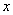## Solving Ordinary Differential Equation (ODE) Numerically

Though we can solve most of ODE using calculus, sometimes, we need the numerical solution within a range of domain. To solve the ODE numerically, the most widely used solution is using 4-th order Runge-Kutta method.

To be able to compare the solutions, in this tutorial I provide the formulation and examples in spreadsheet from the 1 st Order Runge Kutta method (which is famous with name Euler method) up to 4 th order Runge Kutta.

Preferable reference for this tutorial is

Teknomo, Kardi (2015) Solving Ordinary Differential Equation (ODE). https:\\people.revoledu.com\kardi\tutorial\ODE\